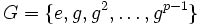# No proper nontrivial subgroup implies cyclic of prime order

Jump to: navigation, search

## Statement

If a nontrivial group has no proper nontrivial subgroup, then it is a cyclic group of prime order. In other words, it is generated by a single element whose order is a prime number.

## Converse

Further information: Prime order implies no proper nontrivial subgroup

The converse is clearly true from Lagrange's theorem. Namely, if we have a cyclic group of prime order$p$, any subgroup must have order either 1 or$p$, by Lagrange's theorem. The only possible subgroup of order$p$ is the whole group, and the only possible subgroup of order 1 is the trivial subgroup.

Note that this also shows something stronger: any group of prime order, since it has no proper nontrivial subgroup, must be cyclic of prime order.

## Proof

Given: A nontrivial group$G$, such that the only subgroups of$G$ are the trivial subgroup, and$G$ itself

To prove: There exists an element$g \in G$ such that$\langle g \rangle = G$, and the order of$g$ is a prime number. In particular,$G = \{ e, g, g^2, \dots, g^{p-1} \}$ with$g^p = e$

Proof: Since$G$ is nontrivial, there exists$g \ne e$ in$G$. Then, consider the subgroup generated by$g$. This is a nontrivial subgroup, hence, by assumption,$\langle g \rangle = G$.

Now there are two possibilities. First, that$g$ has infinite order: no positive power of$g$ is trivial. In this case, the group$G$ is isomorphic to (i.e., can be identified with) the group$\mathbb{Z}$, under the identification$n \mapsto g^n$. In particular, the subgroup generated by$g^2$, which corresponds to the even integers, is a proper nontrivial subgroup, leading to a contradiction.

Hence,$g$ cannot have infinite order, so let$n$ be the order of$g$. Then,$g^n = e$. Suppose that$n$ is composite. Then,$G$ is isomorphic to the cyclic group of order$n$, under the identification$a \mapsto g^a$. Pick a positive integer$d \ne 1,n$ such that$d | n$. Then the subgroup generated by$g^d$ is a proper nontrivial subgroup of$G$ (corresponds to the multiples of$d$ mod$n$). More precisely, it is a subgroup of order$n/d$, because the order of$g^d$ is$n/d$. This is again a contradiction.

Hence,$n$ must be a prime, so$G$ is cyclic of prime order (as desired).